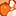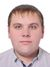# App Development

Discussion board where members can learn more about Qlik Sense App Development and Usage.

Announcements
WE ARE LISTENING! New Navigation for Qlik Community, Sept. 26: TELL ME MORE
cancel
Showing results for
Did you mean:Creator

## Qlik Sense - Conditional Hide / Show Measures in Pivot Table

Hi,

Sample file is attached, please have a look into and suggest me the solution.

if(Sum(total <Dim1> {\$<[Field 1]={1}>} [xxx]) < 0, 1, 0))

I am using pivot table and I would like to Hide / Show the measure when the total value is in Negative. There are two dimensions in the Pivot Table.

When I am writing given above expression in Qlik Sense > Measures >  "Show column if" then it is not working and row is not hiding.

How to fix it?

Labels (4)

• ### Qlik Sense Table

15 RepliesCreator III
You can use below expression as measure:

if(GetSelectedCount(myFilter)>0 and sum(total<Name,Product>{<Name={"=sum(aggr(sum(Sales),Name))<0"}>}Sales)<0,
sum({<Name={"=sum(aggr(sum(Sales),Name))<0"}>}Sales),

if(GetSelectedCount(myFilter)=0 ,sum(Sales)
))Creator
Author

Same result as I shared the expression with this thread.

I need expression to hide and show the row expression "Show Column if"Contributor III

I make use of the Show column if setting of a dimension.

In my case I defined a variable vShowFieldPlantCostCentre with an initial value as '0' (hidden).

Then we use the Variable Input bundled extension, where the user selects from a dropdown box whether to show the column or not. The variable input dropdown box toggles the \$(vShowFieldPlantCostCentre) variable between '0' and '1'.

The Show column if expression is:

`=\$(vShowFieldPlantCostCentre) = 1`

So if the vShowFieldPlantCostCentre variable is equal to '1' then the column will show in the pivot table.

Note that the variables are reset to their default values when the model reloads. So if the model reloads the user will see that his selections (which are linked to variables) revert back to their default values, and in this case causes this column selections to be reset.Creator
Author

Hi Mynhardtb,

I hope, you read the question. However, here is quick overview of problem.

For example: I have Pivot table with SUM Expression. When it execute expression will evaluate and generate the rows for dimension. Now, I have 10 rows 5 consist on Negative (-) and 5 Positive (+) value. I would like to hide and show the Negative (-) rows when select filter "Negative Value".

Expression and Application is already attached with this thread.Contributor III

Hi

See the attached file for 2 possible solutions.

In both cases you will use set analysis to filter for rows where [Sales] is negative like so:

`Sum({<[Sales]={"<0"}>} [Sales])`

I suspect the first solution where you use PICK to select between a SUM which adds up all [Sales] values, and one which only adds up negative [Sales} values is what you are actually after.

I used PICK because it has some performance benefits over using IF statements.Contributor II

Hello)
Thanks a lot for the solution. Changed it a little, instead of expanding, I used the function of the standard button "Assign value to variable")